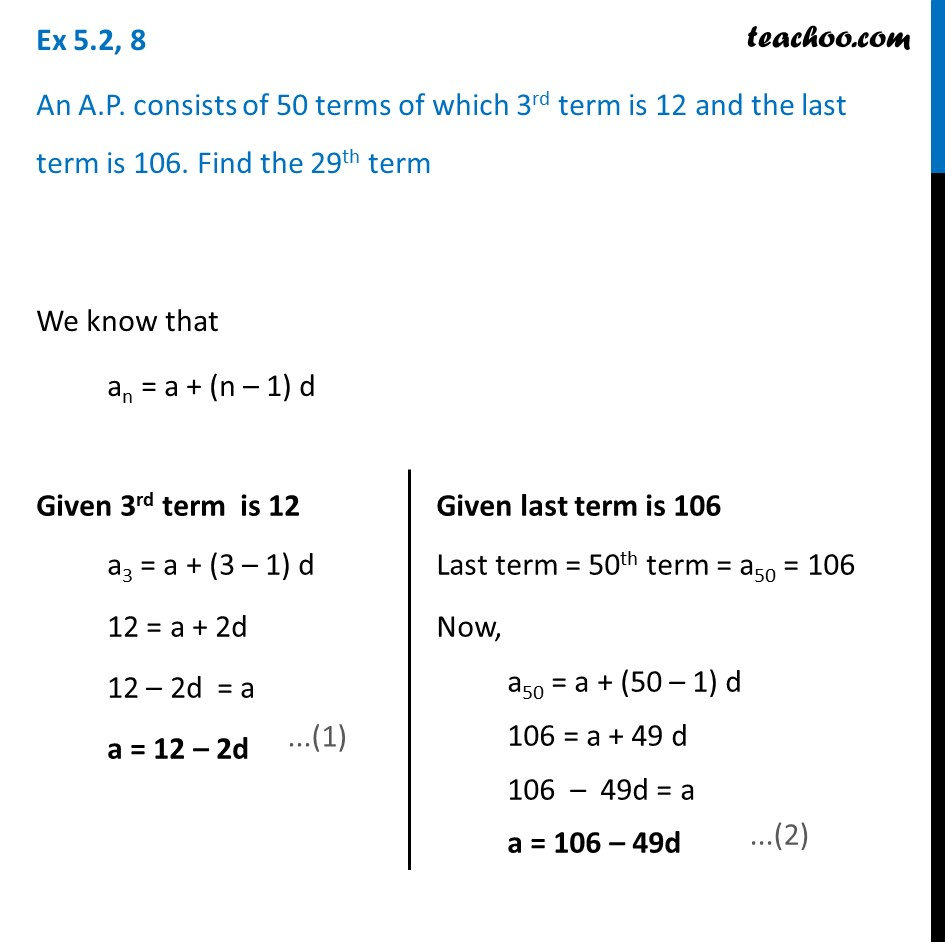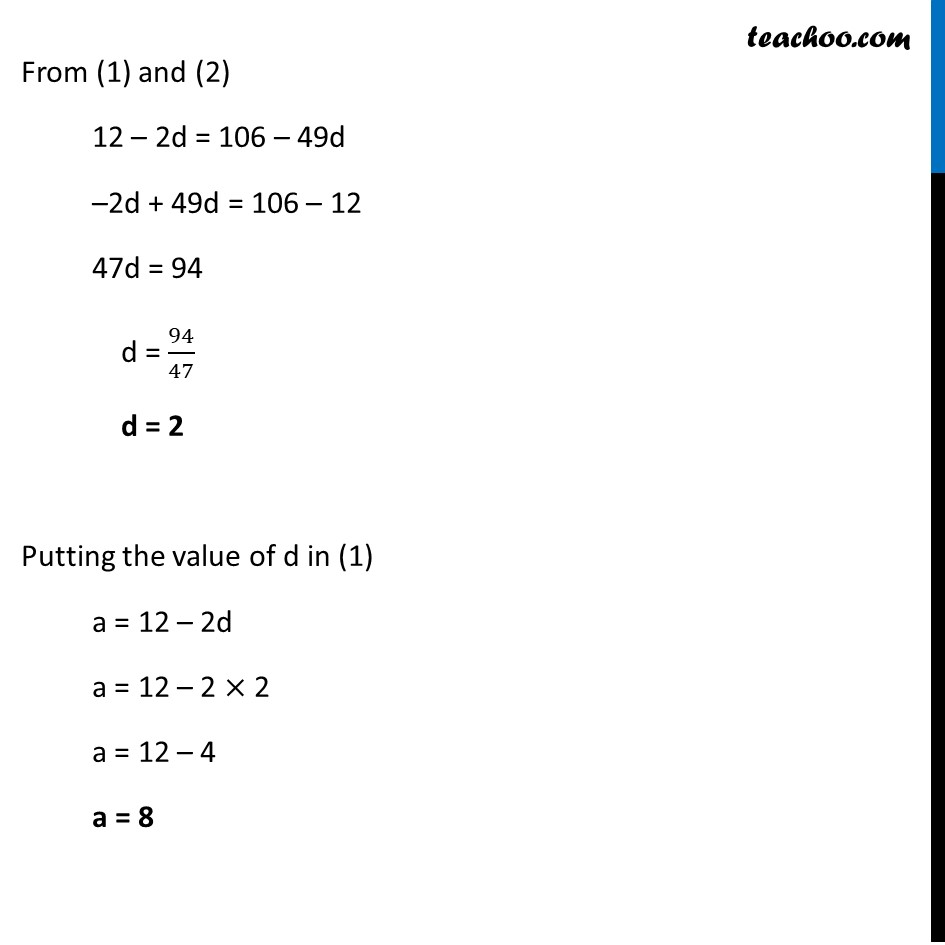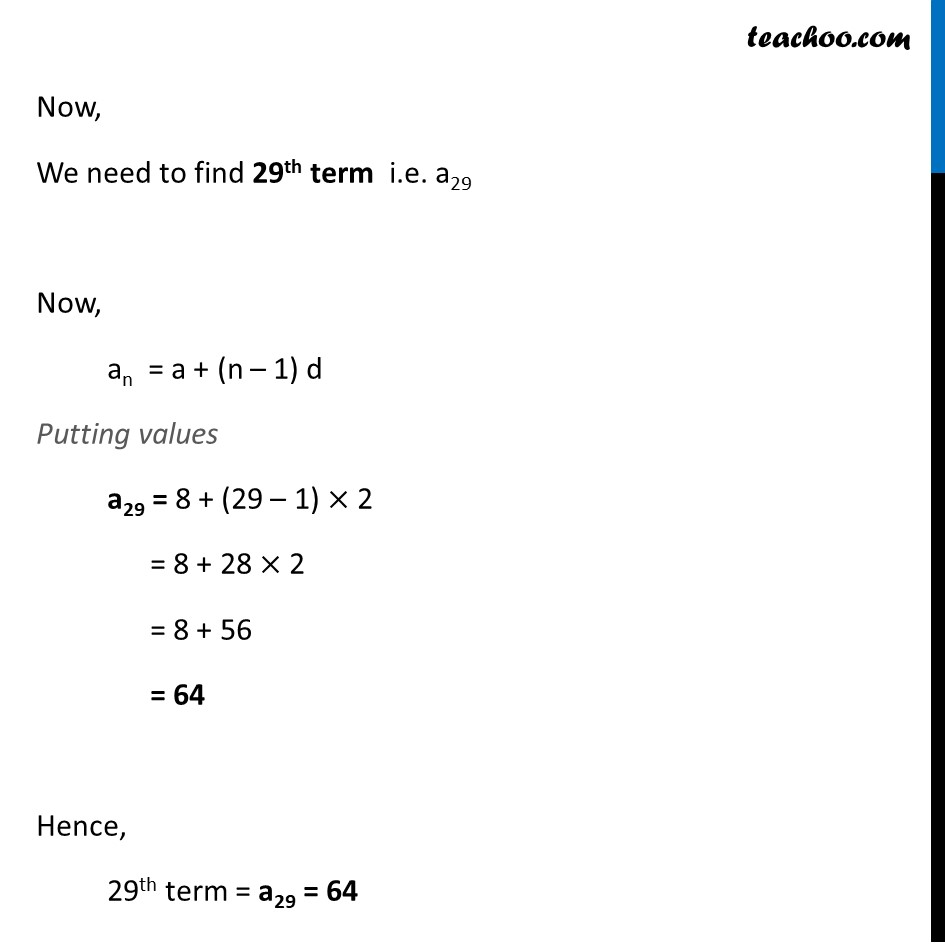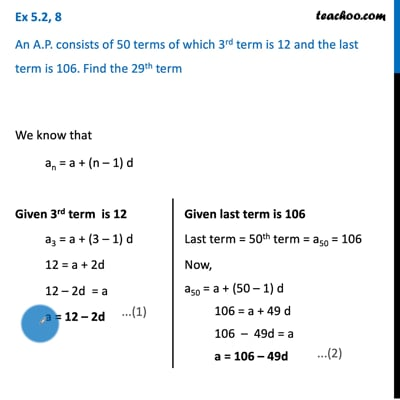Ex 5.2

Chapter 5 Class 10 Arithmetic Progressions (Term 2)
Serial order wiseThis video is only available for Teachoo black users

### Transcript

Ex 5.2, 8 An A.P. consists of 50 terms of which 3rd term is 12 and the last term is 106. Find the 29th term We know that an = a + (n – 1) d Given 3rd term is 12 a3 = a + (3 – 1) d 12 = a + 2d 12 – 2d = a a = 12 – 2d Given last term is 106 Last term = 50th term = a50 = 106 Now, a50 = a + (50 – 1) d 106 = a + 49 d 106 – 49d = a a = 106 – 49d From (1) and (2) 12 – 2d = 106 – 49d –2d + 49d = 106 – 12 47d = 94 d = 94/47 d = 2 Putting the value of d in (1) a = 12 – 2d a = 12 – 2 × 2 a = 12 – 4 a = 8 Now, We need to find 29th term i.e. a29 Now, an = a + (n – 1) d Putting values a29 = 8 + (29 – 1) × 2 = 8 + 28 × 2 = 8 + 56 = 64 Hence, 29th term = a29 = 64